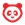cancel
Showing results for
Did you mean:

# How do I create a Formula question in New Quizzes?

## How do I create a Formula question in New Quizzes?

When creating an assessment, you can create a Formula assessment question in New Quizzes. In a Formula question, students need to type in the correct numerical answer.

You can also duplicate Formula questions to create a new question.

Note: View information about Canvas Formula Quiz Question Helper Functions.To create a question, click the Add button anywhere in the assessment.## Add Question Title and StemIn the Question Title field , add a question title. Question titles are optional and are not visible to students.

In the Question Stem field , add the question stem. The question stem contains the question and, if necessary, additional instructions and information. You can use the Rich Content Editor to format and add content to your question stem.

Identify variables in your question by surrounding variable names by backticks. (e.g., a variable name of x can be identified by typing `x`.)After typing the question with the variable, parameters to define the formula will display . Set the variable answers for the formula. Specify the minimum  and maximum  values for the variable. Select decimal places in by using the increase or decrease arrows .Type the formula definition in the formula field. Make sure the formula does not contain any brackets or equal signs.

Notes: The Formula Definition supports the following operators: + (add), - (subtract), * (multiply), / (divide), and ^ (power).

## Generate Possible SolutionsSpecify the number of possible solutions you want to generate in the Number of solutions field . Then select the number of decimal places required in the Decimal places field .

In the Margin type field, select the absolute or percent margin type . Enter the margin of error for the student's answer in the +/- margin of error field . Responses are based on relative error in addition to absolute error. Margins of error can be created as a percentage or as a point value (up to three decimal places).

To display solutions in scientific notation, click the Display as Scientific Notation button .

To view possible solutions, click the Generate button .

## View Possible SolutionsPossible solutions will process according to the number of value combinations.

## Set OptionsTo allow students to use an on-screen calculator for this question, click the Show on-screen calculator checkbox .

If you select this option, you can enable a basic calculator  or scientific calculator . The basic calculator displays numbers and basic math functions (add, subtract, multiply, divide). The scientific calculator displays more options for advanced calculations.

Note: The on-screen calculator option is intended to be used while displaying one question at a time. If the assessment does not display one question at a time, students may be able to use the calculator for all questions.

## Align to OutcomeTo align the question to an outcome, click anywhere in the Align to Outcomes section.If you want to save a question to use in other assessments, you can add it to an item bank.

Note: After a question has been added to an item bank, it can only be edited in the item bank. You can still set unique point values and options in the assessment for an item bank question.To customize the point value for the question, type the point value in the Points field  or use the arrows to increase or decrease the point value .

## Save QuestionTo save the question, click the Done button.

Version history
Revision #:
12 of 12
Last update:
yesterday
Updated by: